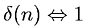Equations > Signal Processing > Z Transform Pairs > Z-transform of delta

### Z-transform of deltaLatex Code:

MathML Code:

 $\delta \left(n\right)⇔1$

MathType 5.0: